## General Mechanical

Topics relate to Mechanical Enterprise, Motion, Additive Print and more

•jacks3215
Subscriber

I would like to carry out simulation of fiber reinforced concrete material under three point bending load in Ansys workbench. Since concrete is a brittle material therefore addition of fibers alters the brittle nature of concrete to ductile. I have already carried out the experimental tests and would like to verify the results with that of finite element simulation.

I would like to request the experts here to guide me on how can I model the random distribution of fibers in Ansys workbench? Since in reality the fibers are randomly distributed with in the given block or space therefore I need to process the same in simulation work.

Thank you

•peteroznewman
Subscriber

There are two kinds of simulations that are possible, but they have different objectives.

One kind is an extremely detailed model that tries to replicate the physics of concrete fracture, fiber breakage, fiber-concrete bond failure, fiber friction, etc to model at a very fine level what is happening to the components in the material under load. The model tries to predict what the experimental data will be, even before it is measured. This is the type of simulation a material researcher might build.

Another kind of simulation is much simpler, taking an existing mathematical material model with some adjustable parameters, and attempting a best fit of those parameters to the experimental data of a three point bending test, so that other more complicated shapes of reinforced concrete structure can be modeled and predictions made for the load carrying capability of those shapes that were never tested. The model of the three point test is going to reproduce the previously measured three point experimental data when the values of the parameters have been properly chosen. This is the type of simulation a structural engineer might build.

Which type of model are you trying to build?

If it is the the second type, then there are several plasticity models that have parameters you can adjust to match your experimental data.

•jacks3215
Subscriber

I am a research student and simple verification of experimental results would be fine.

I also intend to simulate single fiber pullout from the concrete specimen. I have the specifications of concrete, fiber and interface parameters (chemical bond, frictional bond). These parameters I have taken from pullout experiments. From the simulation I could verify the experimental results. If these can be joined together in to the bending simulation, like the first way you mentioned in your answer, then is also ok for me.

For the bending I intend to carry out two simulations, with and without the addition of fibers. Since concrete without fibers will have brittle fracture therefore I could have the maximum strength and the crack and crush from the simulation. With the addition of fibers there will have different behavior resulting in multiple cracks and could have bendable concrete until fracture.

All of my concrete designs are simple, rectangular beam for bending, cubic block for compression. I wont be using any of the complicated structural shapes/designs in my experiments or simulations.
•peteroznewman
Subscriber

Jack, you may find some of my posts on this site relevant.

Here is a simulation of pullout of ribbed rebar from concrete and here is the question about the pullout force result.

Here is a simulation of compression failure of a concrete cylinder without rebar and the post below is with rebar.

Those simulations were using Explicit Dynamics models and are more for post failure illustration than calculating results.

There are many material models available in ANSYS to predict the load when failure would occur, without actually seeing the post failure behavior.

•jacks3215
Subscriber

Thank you for the links. I will follow the links and get back in case of any problem.

I would like to confirm, please correct me if I am wrong, in case I model three point bending simulation of concrete (without reinforcement i,e, brittle fracture), static structural model would be a better choice.

But if I try to carryout three point bending simulation of fiber reinforced concrete in static structural then will the static structural be able to get the post cracking behavior as well? or I must use explicit dynamics for such purpose?

In addition, could you please guide me how could I model fibers random distribution with in a concrete block in Ansys workbench?

•peteroznewman
Subscriber
•peteroznewman
Subscriber

Attached is a zip file with an Excel spreadsheet, a text file and an ANSYS 18.2 project.

The spreadsheet creates 100 randomly oriented straight fibers with a random length between 0 and 5 mm, randomly distributed in a volume of 15 x 25 x 55 mm. You could modify the spreadsheet to create fibers of a minimum length.

The text file is a copy paste of columns O-S of the spreadsheet, which is read into DesignModeler.

After reading that file in and creating a volume, you can see the result shown in the image below.

This is not a complete solution, just an example of how to create random line bodies in DesignModeler.

I have used line bodies as steel rebar to reinforce concrete columns in Explicit Dynamics. Not sure how to use this in Static Structural.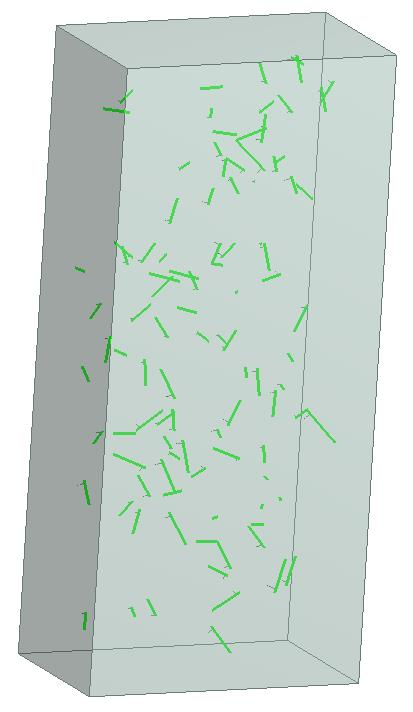•jacks3215
Subscriber

Thank you for sharing the files. With reference to the attached files I would like to ask following queries,

I will be drawing the mold size to 400x400x1600 mm. I can assume that fibers are straight. I would like to ask is it possible to keep the fiber length constant at desired value, say 12 mm? Instead of using random length between 0-12 mm? With in the experimental work I have kept fiber length constant so if the values in simulation are different/varying then I might get different result in simulation.

I have checked values of the text file and the Excel spreadsheet, columns from O to S, but the values of the columns Q-S differ from those mentioned in the text file. Could you please re-check this?

Thank you

•peteroznewman
Subscriber

I chose to randomize the fiber lengths because it was easy to do.  I can randomize the orientation of fixed length fibers, I just have to do a bit more math. This is all being done outside ANSYS using functionality in Excel.  The reason the text file and the Excel spreadsheet are different is because each time you open Excel, it creates 600 new random numbers to fill the cells.

There is more work to be done to figure out how to have the fibers bond to the concrete in a Static Structural model. I only know it is simple to do in Explicit Dynamics.

I will attach an Excel file example with the 12 mm fibers once I have got the equations worked out.

•peteroznewman
Subscriber

Attached is a zip file with an Excel spreadsheet to calculate the text file used in the ANSYS 18.2 model with 12 mm long randomly positioned and oriented fibers. I only put in 49 fibers and the solid because there is a 50 body limit on the Student license.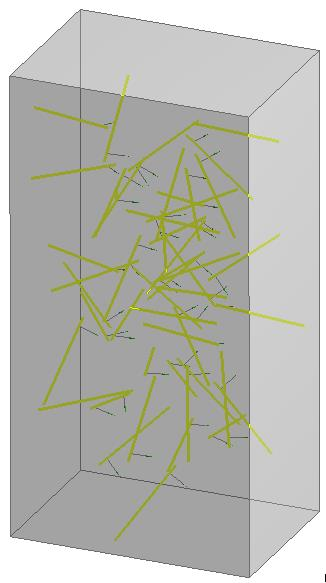•jacks3215
Subscriber

Is it possible to set the fiber diameter? I will be using around 35-39um for different simulations/results.

Also, since in experimental work I have used fibers by volume percentage by weight, around 2-3%. (I can also mention the fibers weight here used for different tests according to the fiber volume percentage. How can I control the fiber quantity in terms of volume percentage if I could access the ansys workbench  pro version?

•peteroznewman
Subscriber

Fibers can be assigned a circular cross section. With a 35 micron diameter and a 12 mm length, I can calculate the fiber volume. If I divide 3% of the beam volume you provided above by the fiber volume I come up with 665 million fibers. Is this what you expect?  When you say by volume, what is the packing density of the fibers? Perhaps it is more realistic to go by weight. What is the density of the fiber material? With that data, I can calculate the number of fibers by weight.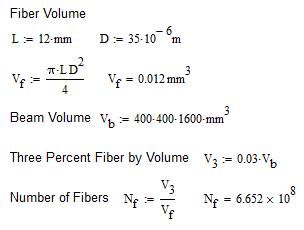Excel can only hold 1 million rows, so you will have to write a program to generate more than a million rows in the text file.

I'm concerned that ANSYS will not be able to handle millions of fibers. Maybe it can. I don't know.

You can cut the size of the model by a factor of 2 by using symmetry to model only half the width. You could get another factor of 3 reduction by only adding fibers to the center 1/3 of the beam where the failure will occur, and having no fibers in the end thirds where there will be no failure.

•jacks3215
Subscriber

The fiber density is 1.3 gm/cm^3 while as for the 2% volume percentage for bending beam (40*40*160 cm) I have used around 6.7 gm of fibers. I agree with your suggestion to use symmetry to model half the width, if the number of fibers are exceeding the limit of the Ansys  or Excel software.

With the addition of fibers there will be multiple cracking instead of a single large crack. If there are constraints then maybe, as you have said, for the three point bending I could add symmetry at the center and only model the fibers near the loading area i.e. around center of the beam.

Apart from this, I have seen some reference papers in which people have carried out fibers random distribution. If you can provide me your email address I can share the reference papers with you for further deep discussion.

Thank you

•peteroznewman
Subscriber

Jack,

Your numbers don't work out for fiber density since 2% of the volume of the beam is 129 cm^3 and you are adding 6.7 g of fibers. That means the fiber density is 6.7/129 = 0.052 g/cm^3, not the 1.3 g/cm^3 you say above. One way both of these numbers can be correct is if the fiber itself is 1.3 g/cm^3 but when you have a container of fibers, they only have a 4% packing efficiency. In other words, there is 96% air in the volume occupied by the fibers. Please clarify as your answer has a big effect on the number of fibers that should be in the beam.

From this reference...

The generation of the RVE is usually realized through the random sequential adsorption (RSA) algorithm . Based on this algorithm, researchers attempted to develop efficient approaches for determining whether a new generated fiber overlaps with the pre-existing fibers within the RVE

The Excel spreadsheet I created above positions fibers in the volume randomly, without regard to whether they overlap or not. It seems that past researchers were using an RSA algorithm to avoid fiber overlap. What are your thoughts on needing to implement some check on fibers that physically occupy the same space?

•jacks3215
Subscriber

I am sorry for mistyping the values of the beam mold, the correct beam size is as follows,

beam size in mm = 40*40*160

beam size in cm= 4*4*16

The fibers density itself is 1.3 gm/cm^3. The way I calculated weight of fibers is as follows,

beam volume in cm^3 = 4*4*16

fiber volume % = 2

weight of fibers = beam volume in cm^3 * fiber volume percentage * fiber density in gm/cm^3

= (4*4*16) * (2/100) * (1.3)

The resultant we will get would be 6.7 grams.

With reference to the correct size of the beam, could you please modify the excel file? It would be useful if we don't have higher number of fibers problem as before.

With regard to the RSA algorithm, it would be useful if such algorithm can be implemented in Ansys WB. I can have a try with simple excel file, but since, as you have also mentioned that researchers have used RSA algorithm for such purposes, I feel it would be a better choice to have a check on fiber placements.

•peteroznewman
Subscriber

Jack, we need the number of fibers to make the Excel spreadsheet.

How many fibers are there is that 2% volume?  Below is my calculation.

The mass calculated for the fibers doesn't match the 6.7 grams you calculated.
Please calculate the number of fibers in the beam.

If you want to create fibers that do not overlap, please provide details on the RSA algorithm.

•jacks3215
Subscriber

In continuation of my previous calculation, the way I carried out calculation for number of fibers is as follows,

No. of fibers = total fibers weight / single fiber weight

No. of fibers = total fibers weight /(fiber length*fiber diameter*fiber density)

No. of fibers = 6.656/(1.2*0.0035*1.3) = 1219

I am sorry, but within your calculation I could not get why and how you calculated the V02 (2% Beam volume). In my opinion, beam volume will be constant (40*40*160 mm^3) no matter how much volume fraction of fiber reinforcement we will be using. Apart from this, if you have any reference/link where such calculations could be found then please mention.

•peteroznewman
Subscriber

Here is your calculation with the correction that the single fiber mass is the length*cross sectional area*density.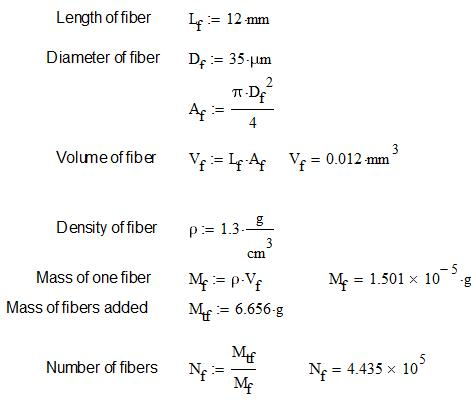In the previous post, I calculate the volume of the beam. Then I multiply that volume by 2% or 0.02 to get the volume of fibers.

•jacks3215
Subscriber

Is it possible in excel to generate the number of randomly distributed fibers (Nf) which you have mentioned in your last reply?

For the RSA algorithm, I have attached an image from the following reference, which we can modify according to our sample size, if there is anything else in RSA then please let me know.

https://www.sciencedirect.com/science/article/pii/S0263822315005097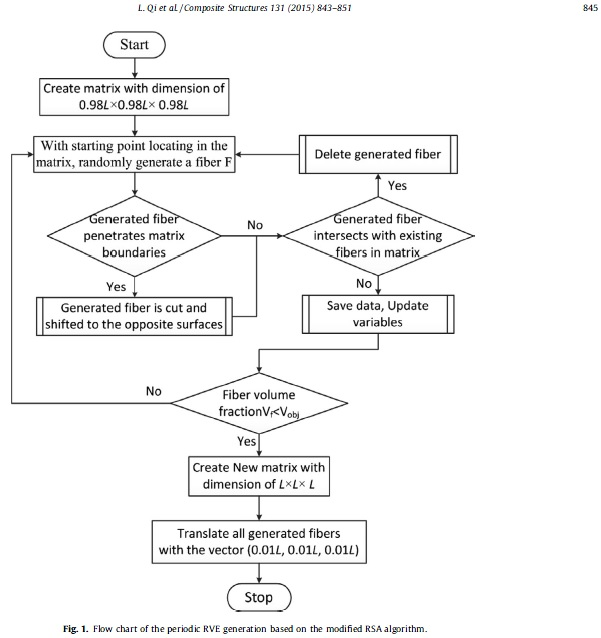•peteroznewman
Subscriber

Excel 2013 limits are 1,048,576 rows x 16,384 columns, so yes, it can be used to generate 443,500 fibers.

However, if you are going to implement the RSA algorithm, you can generate the random fibers in code.

What programming language can you use to implement the RSA algorithm?

The flowchart is very high level, do they have code also?

Can you provide the algorithm and equations to test if Generated fiber intersects with existing fibers in matrix?

Is it appropriate to cut the fiber and shift it to the opposite side when the fiber penetrates the boundary?

•peteroznewman
Subscriber

Can you write the code to detect if these two fibers intersect?

Fiber 1

Start Point     XC =   -5.895375631    YC =    0.198437175     ZC =    6.292249981

End Point      XC =    5.000000000     YC =    4.000000000    ZC =    3.000000000

Fiber 2

Start Point     XC =    0.000000000     YC =    0.000000000     ZC =    0.000000000

End Point       XC =    6.928203230     YC =    6.928203230     ZC =    6.928203230

•jacks3215
Subscriber

Usually Matlab is used for RVE and the resultant is connected via python or excel with simulation softwares. The above mentioned reference does not provide the codings along with it. It will take a while for me to look for the codes and check the intersection which you have mentioned in your reply.

In meantime, if you could help to make the random distribution in excel spreadsheet, and we could run the simulation to check the workability.

•peteroznewman
Subscriber

Do you have access to matlab? If so, I will create a matlab script that generates random fibers in the beam volume and writes a text file for import into ANSYS. No need to use Excel.

•jacks3215
Subscriber

Yes, I have access to Matlab. For the Ansys I can manage to use research licensed version

•peteroznewman
Subscriber

Attached is the matlab code to generate random fiber point coordinates. You can add the code to detect overlapping fibers.

Unfortunately, the limit for bringing in curves through DesignModeler is 2000 curves. This would have to be repeated 217 times to get the full number of curves imported.  I will investigate import into SpaceClaim next.

•peteroznewman
Subscriber

It seems SpaceClaim read all 433,500 curves.

•Ansysman
Subscriber

Please, Sir, can you tell me, how can I use your files 18.2 in my 18.1 version?

•peteroznewman
Subscriber

@Ansysman, version 18.1 cannot open files saved by version 18.2.  However, much of this discussion concerned using tools such as Excel or matlab to create text files to read into Ansys.  You can read those text files into version 18.1.  Do you have matlab?

•Ansysman
Subscriber

Thank you for your quick response!
Not yet, but what version of matlab should I get? Is it ok to use a free matlab trial to solve this problem?

•peteroznewman
Subscriber

You can download the free, open-source software, GNU Octave, which will not expire and runs matlab scripts.

•jacks3215
Subscriber

I tried importing the text file in the ANSYS Design Modeler (DM) but have some issues, something I am doing wrong here needs your guidance.

(1). After creating the beam in DM, goto Concept > 3D Curve > Select 'from coordinates file' in the definition and then select the text file. I followed till here to get the image which I have attached. Two issues here,

(1-a) Please confirm is it the correct way of importing the text file geometry in DM?

(1-b) After importing the axes of the beam and the imported geometry seems to be not the same. The imported geometry should lay inside the beam/solid. As Peteroznewman has shown in his posts. I am missing something here please correct me.

(1-c) After following up to the step (1) mentioned above, when I click Generate I get an error saying,

Error: Reached the maximum number of curves allowed for the curve feature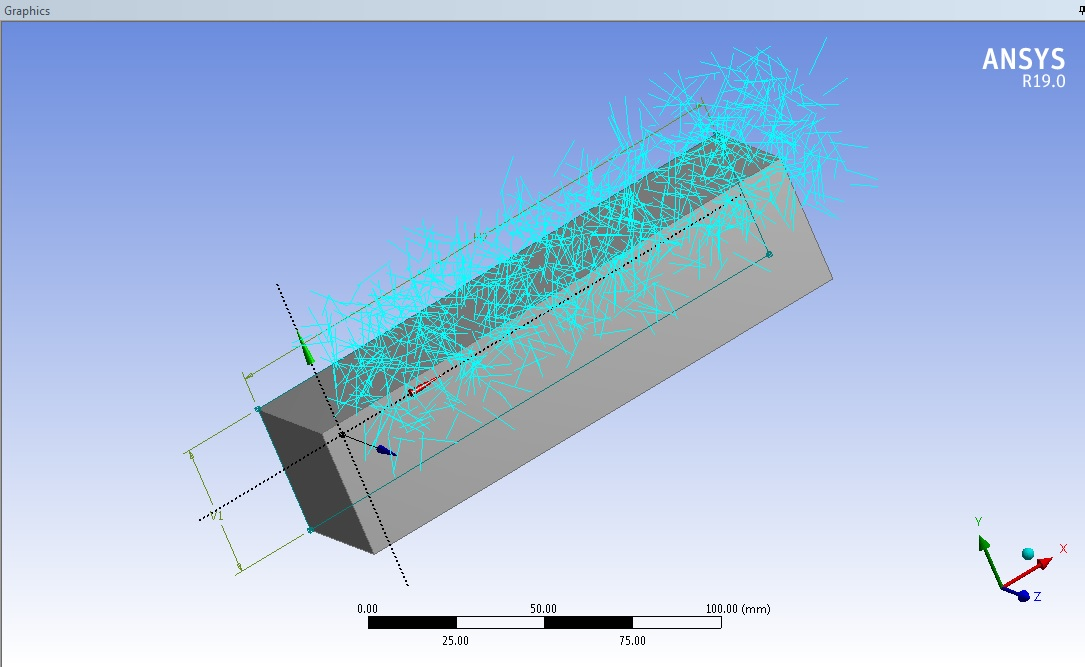Context: Curve Feature Curve2

File: C:.......fibers.txt

Line: 2000

Apart from the above issues. Is it possible to generate an interface around each fiber while generating the randomly distributed fibers? Later, I may define the interface parameters like interface thickness, bonding energy, frictional bond etc.

•peteroznewman
Subscriber

Jack,

(1a) Yes, this is correct.

(1b) When you import, you select a Base Plane. You must select the XY Plane if you want the coordinates in your file to be in the global coordinate system.  Perhaps you had a face or another plane selected when you issued the 3D Curve command.

(1c) The limit for bringing in curves through DesignModeler is 2000 curves. However, you can break your file into multiple files to stay under the limit and import each file individually. SpaceClaim has no limit.

I don't know about generating an interface around each fiber.

•jacks3215
Subscriber

Could you please explain how did you import the text file geometry in the Ansys SpaceClaim?

I have designed a beam in the Ansys SpaceClaim but can not find a way to import the text geometry in to the beam.

•peteroznewman
Subscriber

Go to the Assembly Tab, click on the File icon on the ribbon (not File on the top menu bar). Pick the text file generated by the matlab code I provided above.

That will bring all the curves into the current open SpaceClaim document.

•jacks3215
Subscriber

Got some of the issues, kindly guide me on the following,

(1) I tried using the Matlab files (both SpaceClaim (SC) version and the other one) but for both, I am getting the following error,

Error using  ./

Matrix dimensions must agree.

Error in spaceclaim_random_fiber_generator (line 34)

rne = lf*rne./delta;

Whether or not I change the number of fibers, I still get the same error.

(2) I am using Ansys version 19 and I was unable to open your attached beam model in SC, because of the difference in version.

(3) I created the beam in SC but when I import the geometry (your attached txt file) in the SC, I ended up with the difference in axes orientation problem as shown in the attached image. How can I put the imported geometry and the beam on the same axes?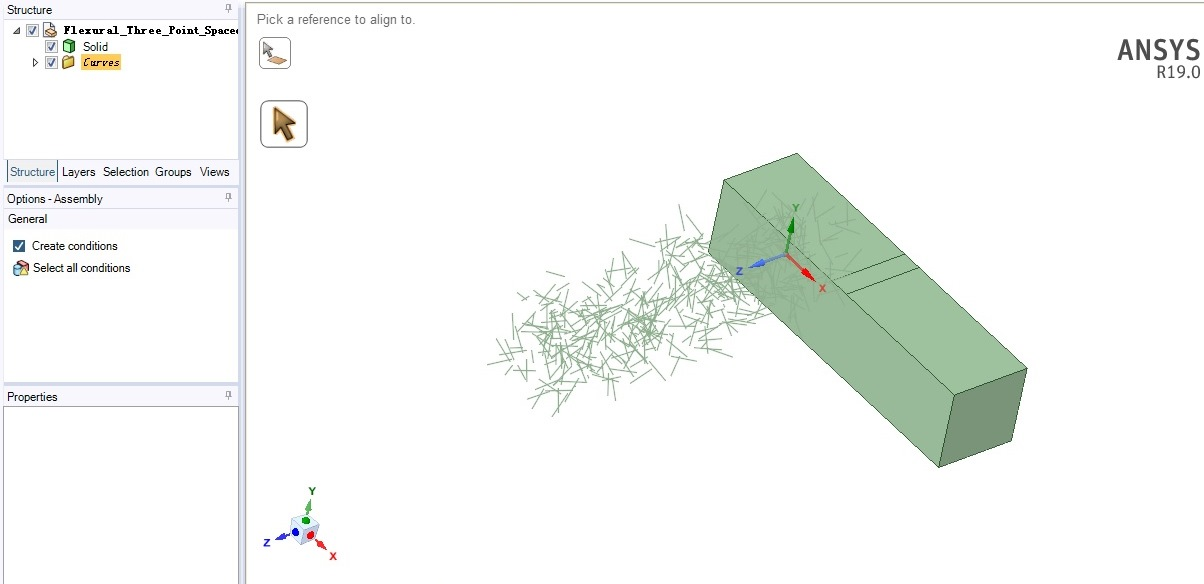•peteroznewman
Subscriber

Jack,

(1) The matlab code I provided runs without error in matlab 2017a.  Are you using matlab or octave?  You can rewrite that line to do the multiplication and division in a for loop instead of the scalar divide shortcut that is not working for you.

(2) I used ANSYS 19.1 so you can't open that in 19.0

(3) In DM, the plane that is selected at the time a file import is done is the "Base Plane" for that data. Maybe SC does the same thing. I don't know. The simple work around is to change matlab to put the long axis of the box in the Z direction instead of the X direction.

% Box dimensions

bx = 40;

by = 40;

bz = 160;

•jacks3215
Subscriber

(1). I ran the code in Matlab 2016a but it did not work. I do not have much knowledge of Matlab codings if you may modify the *.m file to use loop as you have suggested in your reply. However, it is working fine in matlab 2017a. I have checked it.

(3) I modified the box dimensions but got some issues as follows,

(a) Some part of the imported geometry is laying outside the box (form right, top and bottom) as shown in the attached image. I wonder it might affect the simulation outcome. Could we just bound the imported geometry to lay inside the box in SC or modify the *.m file to do so?(b) I tried using the geometry in Mechanical, but the mechanical is only showing the beam or the box, not the imported geometry. After importing the geometry in SC (as shown in the previous image) I saved the geometry file (*.scdoc). Then dragged Static Structural in WB and imported that geometry file and opened the Mechanical. I ended up with the image of beam only. Even the wireframe is showing nothing inside the beam. Maybe I am doing something wrong or our geometry has some issue for Mechanical to load.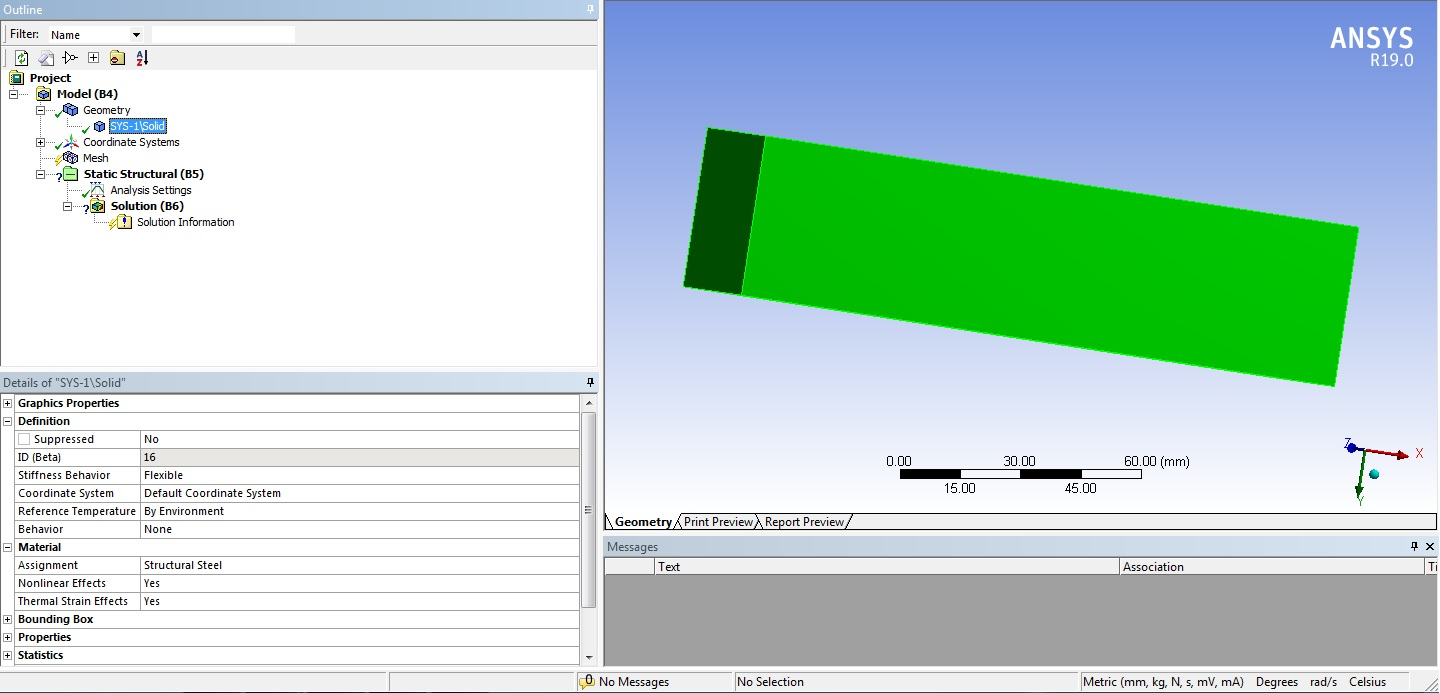•peteroznewman
Subscriber

Jack,

(1) I ran the code in 2016b and it runs.  There must have been a bug in matlab 2016a. Comment out the line that doesn't work and use a for loop shown below (I had to use an image since this site converts some characters into a smiley faceautomatically).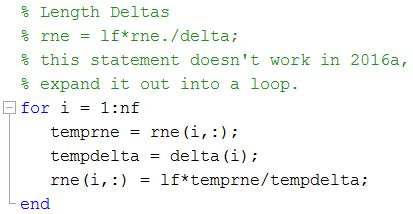I don't want to install 2016a to test it, so you will have to tell me if that runs, or you can upgrade to matlab 2016b or 2017a. Don't forget to manually paste in the two header lines at the top of the text file.

3d=true

polyline=true

(3a) Make the matlab Box dimensions smaller, instead of:

% Box dimensions

bx = 40;

by = 40;

bz = 160;

use Box dimensions that are 12 mm smaller for fibers that are 12 mm long.

% Box dimensions

bx = 28;

by = 28;

bz = 148;

Note that the fibers generated go up to 6 mm beyond the box dimension on all sides. If you don't want any fiber outside the SC box, you must draw your box in SC with an origin of (-6,-6,-6). Alternatively, you could add 6 to the x,y,z coordinates of the start and end points in matlab before writing out the text file, then the fibers would extend up to 12 mm beyond the x,y,z box dimensions in the positive direction and no fiber would have negative coordinates.

(3b) Import created Curves. In SC, you have to generate Beams from Curves. Click on the Prepare tab, and define a cross-section for the beam by clicking on the Profiles pulldown and select Circle.  You will later edit the Circle to type in the diameter you want, but that is a whole new discussion.

You can see the Create Button is now active and a Beam Profiles folder is under the list of Curves. Click on the first curve in the Structure, and shift click on the last curve in the Structure, then click the Create button.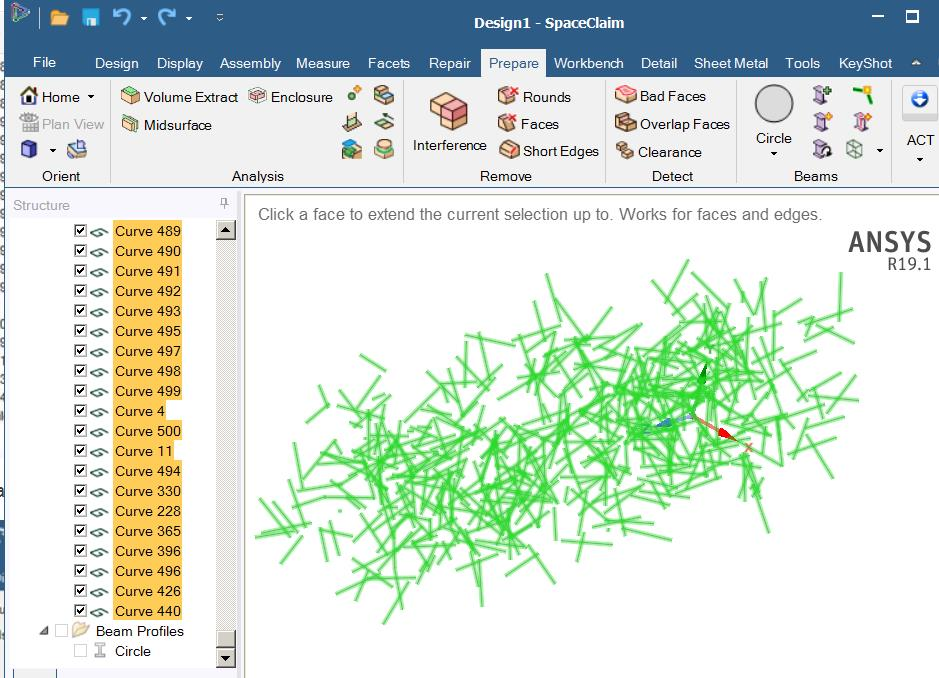All the curves become Beams and the Curves are removed.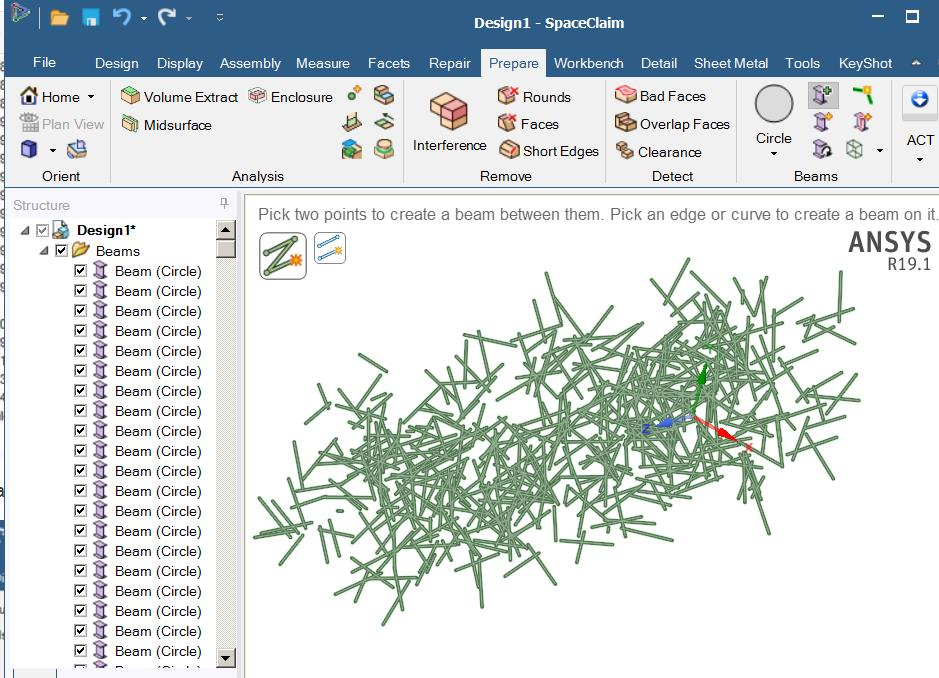Regards,

Peter

•jacks3215
Subscriber

Loop is working fine now in Matlab 2016a version.

•rockmanqh
Subscriber

Dear,

Is there a way to create the mesh from this text file in ANSYS APDL?

Thanks,

•peteroznewman
Subscriber

Dear rockmanqh,

Yes, I am sure there is a way. I don't know APDL, but there are members on this site who are experts.

I suggest you start a New Discussion and post a detailed question with a small example text file in the post.

A new discussion will get a lot more attention than a brief question on the end of a very long discussion.

Regards,

Peter

•sandeep
Subscriber

respected sir,

i have tried to model the composite pipe in the same way as u  have explained on concrete rebar topic and using the excel sheet code i assigned the line bodies a cross section but i am not getting the result the result are not showing any effect of 100 line bodies .

•peteroznewman
Subscriber

Dear Sandeep,

You can copy a #Permalink from any post in this discussion to include a link in your own discussion.

Regards,
Peter

•William
Subscriber

Hello hye. As u mentioned about the fiber orientation above, u assume the fiber is straight right ? how about if the fiber look like a glass giber ? have a curves..my question is, can i still using the same generator random fiber in matlab file that u provide in this case ?

•peteroznewman
Subscriber

No William, you can't use the same generator, but you could add some code to put a third point between the start and end with a random amount of curvature in a random plane along the axis.

•William
Subscriber

Thank you for ur feedback Mr. Peter..Can u provide me a new generator for glass fiber orientation ? This is new knowledge for me. I hope u can help me sir. The dimension of box will be 100mm x 100mm x100mm. Length of fiber is 36mm and diameter is 16µm. So all the fibers should inside of this box.

•peteroznewman
Subscriber

William, build a model with straight fibers first. At the time I wrote this discussion, I did not know how to use the fibers in a model.  Later I learned how and wrote about it in this post.

Do you have matlab?  If not, I tested that my code runs in the free, open-source program called Octave version 5.1.0. Learn to code.

If you have new questions open a New Discussion.

•William
Subscriber

Sir Peter, yes i have Matlab. But im still new with this matlab software. If u dont mind, u cant make a script or any commands for glass fibers orientation.

•peteroznewman
Subscriber

•Maxwell Smith